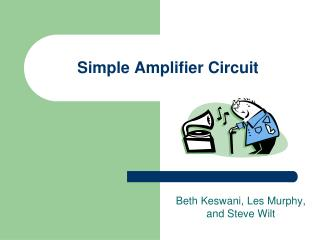DownloadDownload PresentationSimple Amplifier Circuit

Simple Amplifier Circuit

Télécharger la présentationSimple Amplifier Circuit

- - - - - - - - - - - - - - - - - - - - - - - - - - - E N D - - - - - - - - - - - - - - - - - - - - - - - - - - -
Presentation Transcript

1. Simple Amplifier Circuit Beth Keswani, Les Murphy, and Steve Wilt

2. What is an amplifier? • An amplifier is an electronic device that enlarges or extends power, current, or voltage. We are using the amplifier for sound.

3. Amplifiers In Action • Audio amplifiers are used in stereo systems, car systems, and personal radios. Bands also use amplifiers to create a louder sound. I have an amp in me! Whoa! That’s loud!!! This is Les

4. Capacitors Op amp Resistors Wave generator for voltage input Variable Resistor What Do We Need? • Op Amp- LM386 • Variable Resistor- 102 • Capacitors- 10μF and 250μF • Voltage Input- 1KHz • Low Impedence Resistor- 4Ω, 8Ω, 16Ω, 32Ω

5. Basic Schematic Drawing

6. PSpice Schematic Model

7. Whoo Hoo! It worked!

8. How to Read the Results • The yellow wave is the output. • The blue wave is the input. • We can tell this worked because there is a gain, so it shows that the amplitude was increased. • To calculate the gain, we used channel 1 (yellow wave) peak to peak channel 2 (blue wave) peak to peak.

9. The Tektronic Results Are In… • This is using a 4Ω resistor. • The gain is 154.

10. More Fun Graphs… • This is using the 8Ω resistor. • The gain is 205.

11. Keep The Fun Coming… • This is using the 16Ω resistor. • The gain is 170.

12. Aw…the fun is almost over • This is using the 32Ω resistor. • The gain is 189.

13. PSpice and Tektronic Team Up! • These results will show the PSpice results and the Tektronic results compared together. Each PSpice result includes a graph similar to the Tektronic results and also a graph showing the frequency.

14. Using the 4Ω resistor Percent Error is 54%

15. Using the 8Ω resistor Percent Error is 2.5%

16. Using the 16Ω resistor Percent Error is 15%

17. Using the 32Ω resistor Percent Error is 16%